My Blog
Articles related to programming, computer science, technology and research.

23/04/2016 Categories: C Programming. Comments Off on Functions in C

Until now, in all the C programs that we have written, the program consists of a main function and inside that we are writing the logic of the program. The disadvantage of this method is, if the logic/code in the main function becomes huge or complex, it will become difficult to debug the program or test the program or maintain the program.

So, generally while writing the programs, the entire logic is divided into smaller parts and each part is treated as a function. This type of approach for solving the given problems is known as Top Down approach. The top-down approach of solving the given problem can be seen below:Definition: A function is a self contained block of code that performs a certain task/job. For example, we can write a function for reading an integer or we can write a function to add numbers from 1 to 100 etc.

Generally a function can be imagined like a Black Box, which accepts data input and transforms the data into output results. The user knows only about the inputs and outputs. User has no knowledge of the process going on inside the box which converts the given input into output. This can be seen diagrammatically as shown below: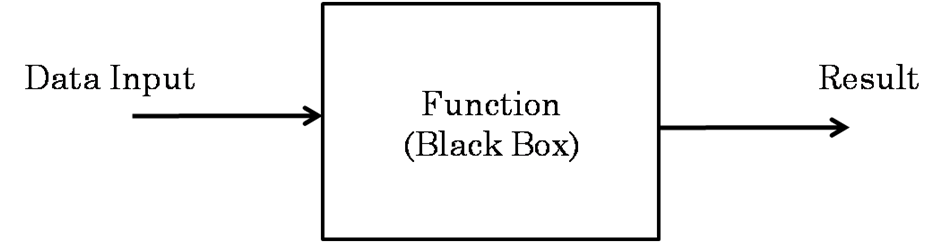## Types of Functions

Based on the nature of functions, they can be divided into two categories. They are:

1. Predefined functions / Library functions
2. User defined functions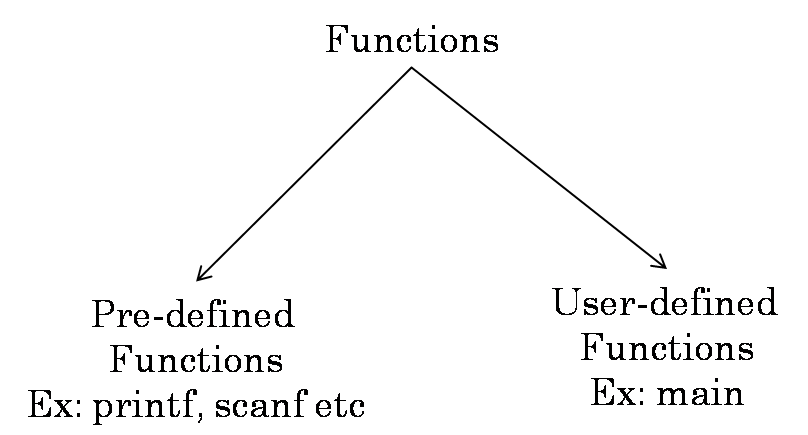### Predefined functions / Library functions

A predefined function or library function is a function which is already written by another developer. The users generally use these library functions in their own programs for performing the desired task.

The predefined functions are available in the header files. So, the user has to include the respective header file to use the predefined functions available in it.

For example, the printf function which is available in the stdio.h header file is used for printing information onto the console. Other examples of predefined functions are: scanf, gets, puts, getchar, putchar, strlen, strcat etc.

### User defined functions

A user defined function is a function which is declared and defined by the user himself. While writing programs, if there are no available library functions for performing a particular task, we write our own function to perform that task. Example for user defined function is main function.

## Need for functions

Functions have many advantages in programming. Almost all the languages support the concept of functions in some way. Some of the advantages of writing/using functions are:

1. Functions support top-down modular programming.
2. By using functions, the length of the source code decreases.
3. Writing functions makes it easier to isolate and debug the errors.
4. Functions allow us to reuse the code..

## Functions Terminology

### Creating functions

For creating functions in C programs, we have to perform two steps. They are: 1) Declaring the function and 2) Defining the function.

#### Declaring functions

The function declaration is the blue print of the function. The function declaration can also be called as the function’s prototype. The function declaration tells the compiler and the user about what is the function’s name, inputs and output(s) of the function and the return type of the function.

The syntax for declaring a function is shown below: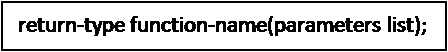Example:In the above example, readint is the name of the function, int is the return type of the function. In our example, readint function has no parameters. The parameters list is optional. The functions are generally declared in the global declaration section of the program.

#### Defining functions

The function definition specifies how the function will be working i.e the logic of the function will be specified in this step. The syntax of function definition is shown below: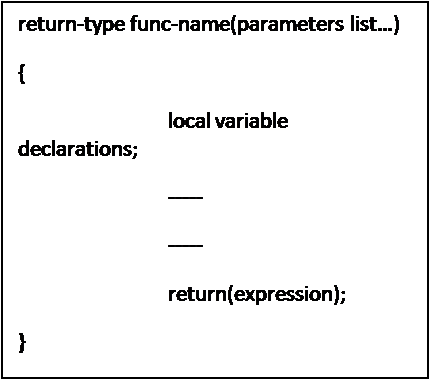In the above syntax the return-type can be any valid data type in C like: int, float, double, char etc. The func-name is the name of the function and it can be any valid identifier. The parameters list is optional.

The local variables are the variables which belong to the function only. They are used within the body of the function, not outside the function. The return is a keyword in C. It is an unconditional branch statement. Generally, the return statement is used to return a value.

Example: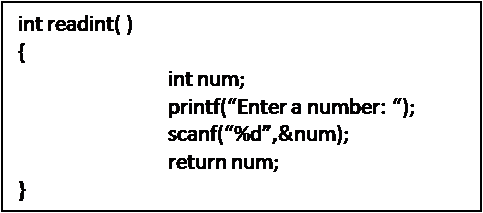In the above example, readint is the name of the function, int is the return type. The identifier num is a local variable with respect to readint function. The above function is reading an integer from the keyboard and returning that value back with the help of the return statement.

Note: Care must be taken that the return type of the function and the data type of the value returned by the return statement must match with one another.

### Using Functions

After declaring and defining the functions, we can use the functions in our program. For using the functions, we must call the function by its name. The syntax for calling a function is as shown below: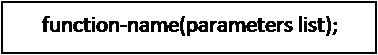The function name along with the parameters list is known as the function signature. Care must be taken that while calling the method, the syntax of the function call must match with the function signature. Let’s see an example for function call:Whenever the compiler comes across a function call, it takes the control of execution to the first statement in the function’s definition. After the completion of function i.e., whenever the compiler comes across the return statement or the closing brace of the function’s body, the control will return back to the next statement after the function call. Let’s see this in the following example: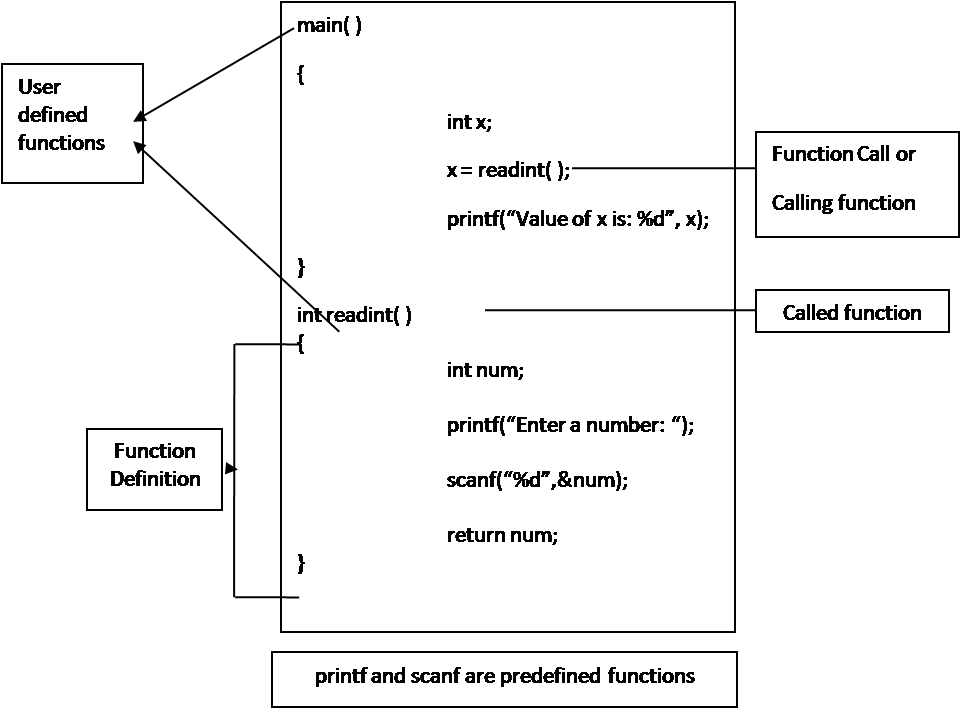### Calling function and Called function

The point at which the function is being invoked or called is known as the calling function. The function which is being executed due to the function call is known as the called function. Example for both calling function and the called function is given in the above example.

### Formal Parameters and Actual Parameters

A parameter or argument is data which is taken as input or considered as additional information by the function for further processing. There are two types of parameters or arguments.

The parameters which are passed in the function call are known as actual parameters or actual arguments. The parameters which are received by the called function are known as formal parameters or formal arguments. Example is shown below: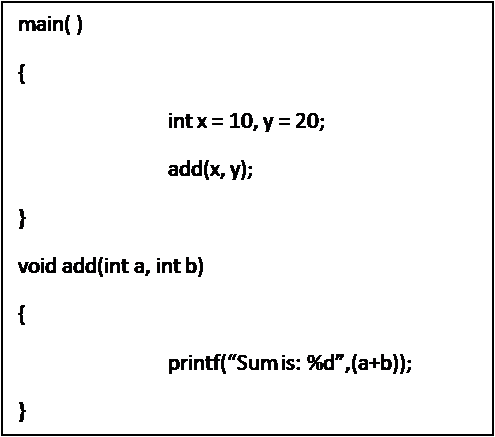In the above example, x and y are known as actual parameters and a and b are known as formal parameters. In the above code, we can see that the return type of the function add is void. This is a keyword in C. The void keyword is a datatype. If the function does not return any value, we specify the data type void. Generally void means nothing / no value.

Note: The formal parameters and actual parameters can have the same names i.e., if the actual parameters are x and y, then the formal parameters can also be x and y. But, it is recommended to use different names for actual and formal parameters.

## Classification of Functions

Based on the parameters and return values, functions can be categorized into four types. They are:

1. Function without arguments and without return value.
2. Function without arguments and with return value.
3. Function with arguments and with return value.
4. Function with arguments and without return value.

### Function without arguments and without return value

In this type of functions there are no parameters/arguments in the function definition and the function does not return any value back to the calling function. Generally, these types of functions are used to perform housekeeping tasks such as printing some characters etc.

Example:

In the above example, printstars function does not have any parameters. Its task is to print 20 stars whenever it is called in a program. Also printstars function does not return any value back.

### Function without arguments and with return value

In this type of functions, the function definition does not contain arguments. But the function returns a value back to the point at which it was called. An example for this type of function is given below:

Example:

In the above example, readint function has no parameters/arguments. The task of this function is to read a integer from the keyboard and return back to the point at which the function was called.

### Function with arguments and with return value

In this type of functions, the function definition consists of parameters/arguments. Also, these functions returns a value back to the point at which the function was called. These types of functions are the most frequently used in programming. An example for this type of function can be seen below:

Example:

In the above example, the function add consists of two arguments or parameters x and y. The function adds both x and y and returns that value stored in the local variable result back to the point at which the function was called.

## Predefined / Library Functions

A function is said to be a predefined function or library function, if they are already declared and defined by another developer. These predefined functions will be available in the library header files.

So, if we want to use a predefined function, we have to include the respective header file in our program. For example, if we want to use printf function in our program, we have to include the stdio.h header file, as the function printf has been declared inside it.

Some of the header files in C are: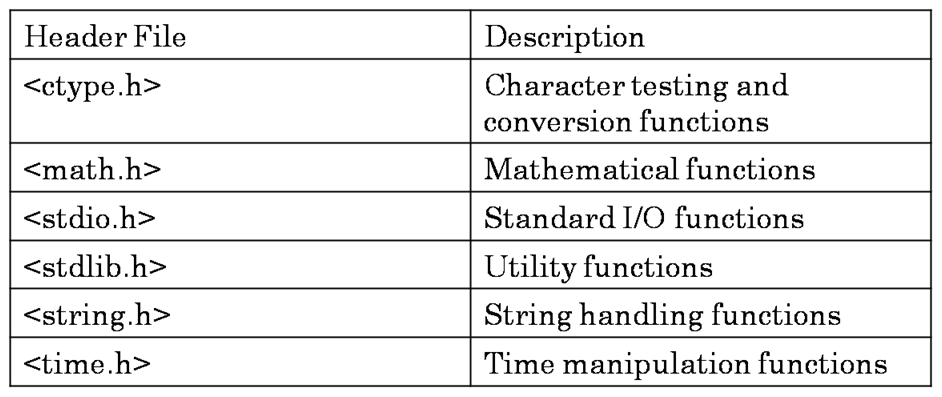Some of the predefined functions available in ctype.h header file are: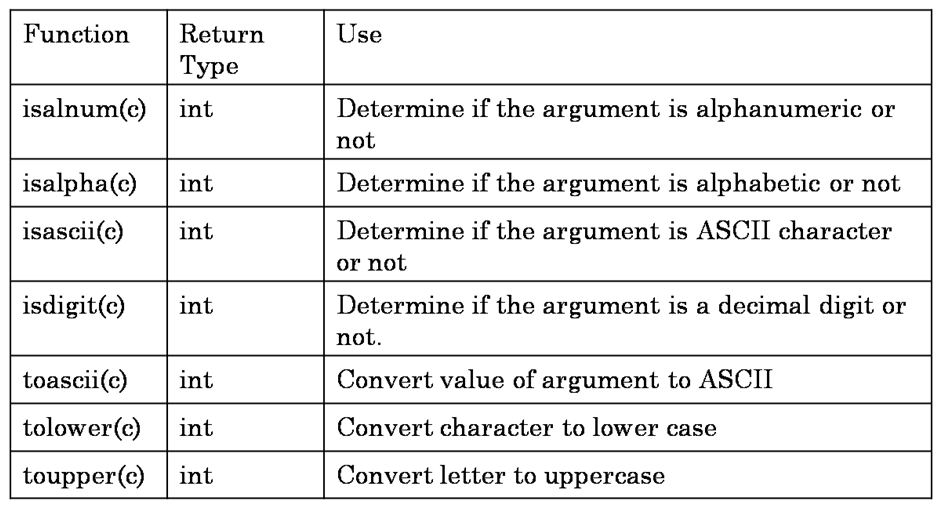Some of the predefined functions available in math.h header file are: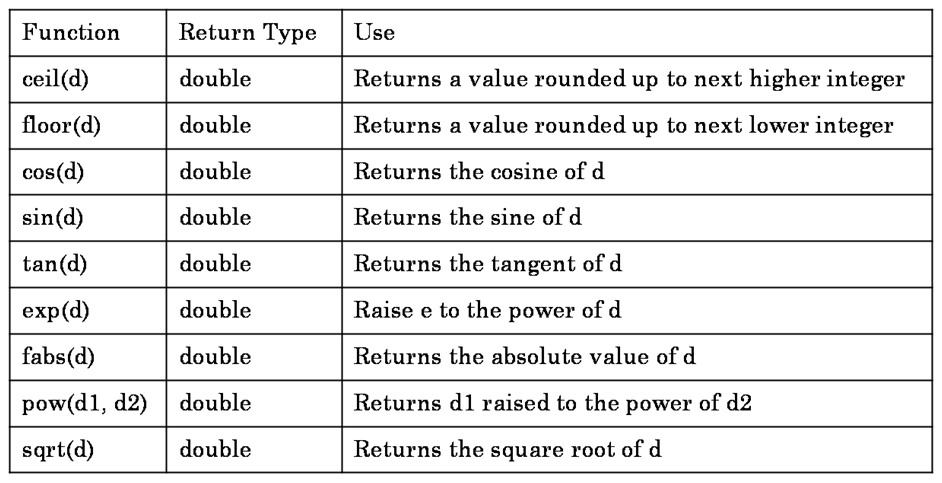Some of the predefined functions in stdio.h header file are: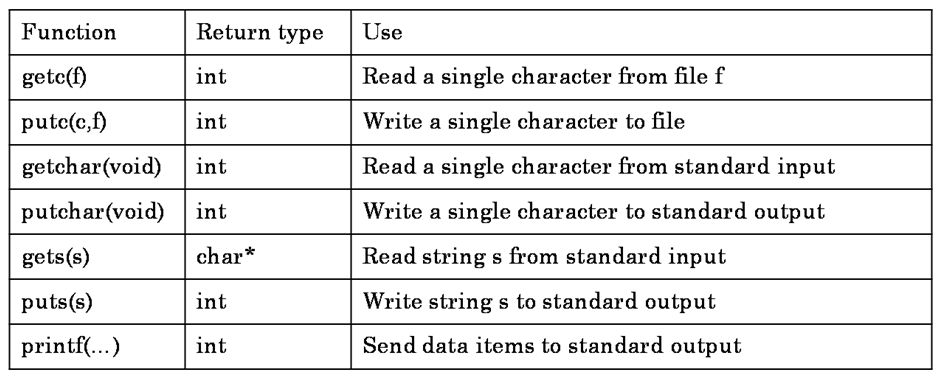Some of the predefined functions in stdlib.h header file are: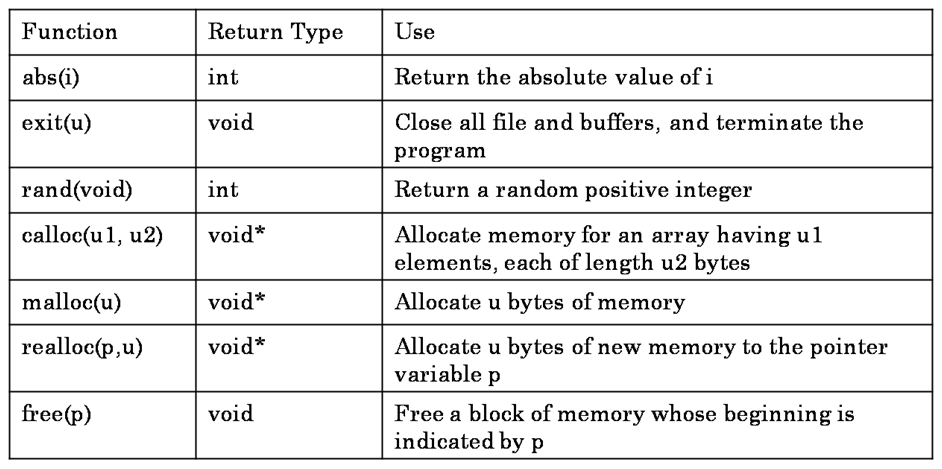Some of the predefined functions in string.h header file are: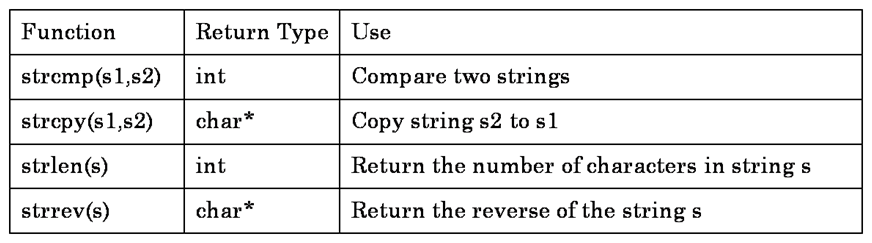Some of the predefined functions available in time.h header file are: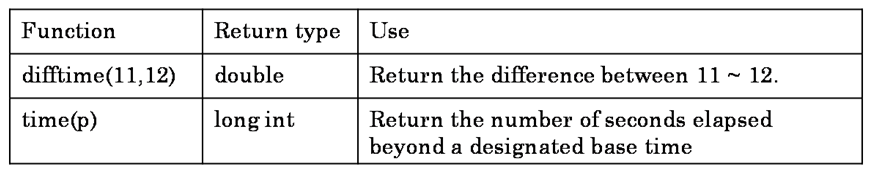## Nested Functions

A function calling another function within its function definition is known as a nested function. So, far we are declaring a main function and calling other user-defined functions and predefined functions like printf, scanf, gets, puts etc., So, main function can be treated as a nested function. Let’s see the following example: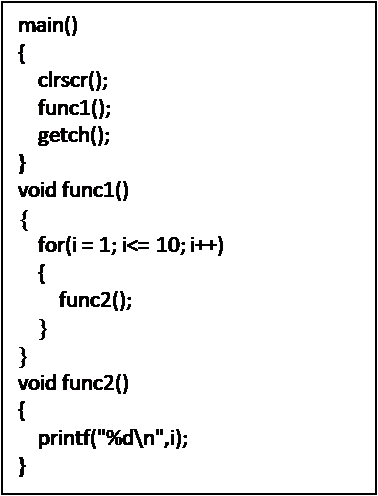In the above example, the main function is calling three functions namely: clrscr, func1 and getch. So, main is a nested function. Also, in the definition of func1, it is calling another function func2. So, func1 is also a nested function.

Note: In programs containing nested functions, the enclosing or outer function returns back only when all the inner functions complete their task.

## Recursion

A function is said to be recursive, if a function calls itself within the function’s definition. Let’s see the following example: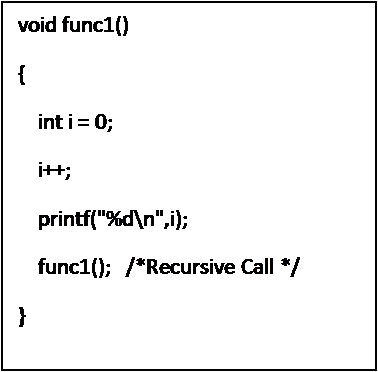In the above example, func1 is calling itself in the last line of its definition. When we write recursive functions, the function only returns back to the main program when all the recursive calls return back.

Note: When writing recursive functions, proper care must be taken that the recursive calls return a value back at some point. Otherwise, the function calls itself infinite number of times.

## Passing arrays to functions

### Passing one-dimensional arrays

We can also pass arrays as parameters to the called function. While passing one-dimensional array to a function, you should follow three rules. They are:

1. In the function declaration you should write a pair of square brackets [ ] beside the name of the array. No need to specify the size of the array.
2. In the function definition you should write a pair of square brackets [ ] beside the name of the array. Again no need to specify the size of the array.
3. In the function call, it is enough to just pass the array name as the actual parameter. No need to write the square brackets after the array name.

When an array is passed as an actual parameter, the formal parameter also refers to the same array which is passed as an actual parameter. When passing an array as a parameter, you are passing the address of the array, not the values in the array.

So, if you make changes in the array using the formal name of the array, the changes are also reflected on the actual array.

### Passing two-dimensional arrays

We can also pass two-dimensional arrays as parameters to a function. While passing two-dimensional arrays as parameters you should keep in mind the following things:

1. In the function declaration you should write two sets of square brackets after the array name. You should specify the size of the second dimension i.e., the number of columns.
2. In the function call you should write two sets of square brackets after the array name. Also you should specify the size of the second dimension i.e., the number of columns.

In the function call, it is enough to pass the name of the array as a parameter. No need to mention the square brackets.